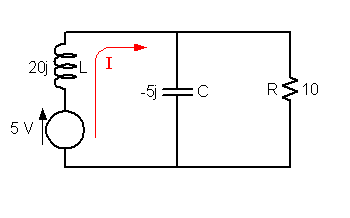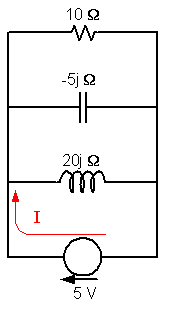# RLC Parallel circuit

## Homework Statement

Hi
Can a kind person please check my solution? This is the question: Demonstrate the total current in an RLC parallel circuit using the following circuit. I cant draw it but the inductive reactance is 20ohms, capacitive reactance is 5ohms and resistor of 10ohms. All are in parallel. The voltage is 5v in series with 20ohms inductive reactance.

## The Attempt at a Solution

This is my attempted solution:
Resistive Branch: I = E/R = 5/10 = 0.5A
Capacitive Branch : E/Xc = 5/5 = 1A
Inductive Branch = E/XL =5/20 = 0.25A
Resultant current, Ix = 1 - 0.25 = 0.75A

Total current = (I^2R + I^2X)^1/2
= (0.75^2 + 0.5^2)^1/2

gneill
Mentor

## Homework Statement

Hi
Can a kind person please check my solution? This is the question: Demonstrate the total current in an RLC parallel circuit using the following circuit. I cant draw it but the inductive reactance is 20ohms, capacitive reactance is 5ohms and resistor of 10ohms. All are in parallel. The voltage is 5v in series with 20ohms inductive reactance.

## The Attempt at a Solution

This is my attempted solution:
Resistive Branch: I = E/R = 5/10 = 0.5A
Capacitive Branch : E/Xc = 5/5 = 1A
Inductive Branch = E/XL =5/20 = 0.25A
Resultant current, Ix = 1 - 0.25 = 0.75A

Total current = (I^2R + I^2X)^1/2
= (0.75^2 + 0.5^2)^1/2

Hello aurao2003.

Is this more or less what your circuit looks like?I suspect that the question wants to know the value of the current I as produced by the voltage source.

Note that since the inductor is in series with the voltage source there will be a potential drop across that inductor before the current reaches the paralleled R & C.

Do you know how to work with impedances (complex numbers)?

#### Attachments

Yes. That's what my circuit looks like. I have done complex numbers in my maths class.

Is my solution on track? Please let me know.

gneill
Mentor
Yes. That's what my circuit looks like. I have done complex numbers in my maths class.

Is my solution on track? Please let me know.

Alas, your solution is not "on track" because you have neglected the potential drop that must occur across the inductor -- the total current passes through the inductor before it ever reaches the capacitor or resistor.

Since you have experience with complex numbers, treat the components if they were plain resistors but with values as shown in the diagram I supplied. What would be the total "resistance" as seen by the voltage source? This "resistance" is the circuit's impedance.

You can find the total current if you have the voltage supplied and the impedance. So first find that impedance.

Alas, your solution is not "on track" because you have neglected the potential drop that must occur across the inductor -- the total current passes through the inductor before it ever reaches the capacitor or resistor.

Since you have experience with complex numbers, treat the components if they were plain resistors but with values as shown in the diagram I supplied. What would be the total "resistance" as seen by the voltage source? This "resistance" is the circuit's impedance.

You can find the total current if you have the voltage supplied and the impedance. So first find that impedance.

Thanks for your help. I mislead you about the diagram. The inductor, capacitor and resistor are all in parallel with the supply voltage at the bottom of the circuit. Does this clarify matters? I apologize for not presenting a diagram.

gulfcoastfella
Gold Member
You didn't provide a frequency for the voltage source. Is the voltage DC or AC?

gneill
Mentor
Thanks for your help. I mislead you about the diagram. The inductor, capacitor and resistor are all in parallel with the supply voltage at the bottom of the circuit. Does this clarify matters? I apologize for not presenting a diagram.

Okay, no problem. So the "new" arrangement looks like this:In this case your original method will work fine. This is because all three passive components are being driven by the same voltage, so you can determine the individual branch currents quite easily. Your answer looks fine.

Note that the complex impedance method would work too (in fact it always works).

#### Attachments

Last edited:
Okay, no problem. So the "new" arrangement looks like this:In this case your original method will work fine. This is because all three passive components are being driven by the same voltage, so you can determine the individual branch currents quite easily. Your answer looks fine.

Note that the complex impedance method always would work too (in fact it always works).

Thanks a million. You are amazing! I might some other. Hope it's okay to let you know.

Cheers!